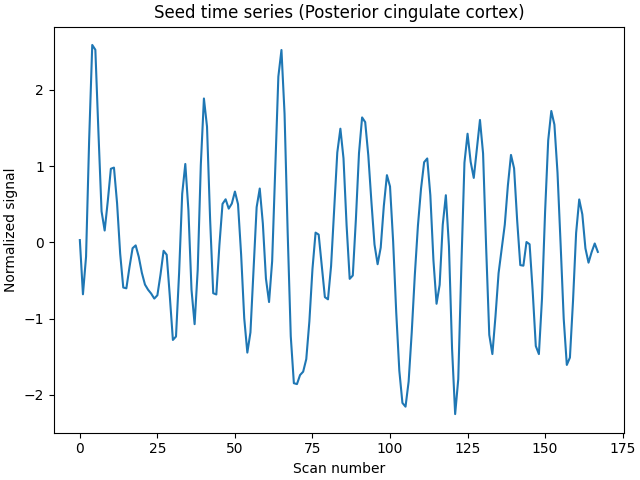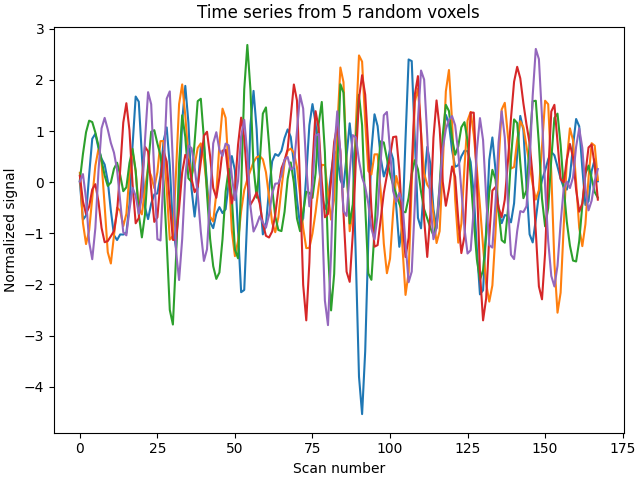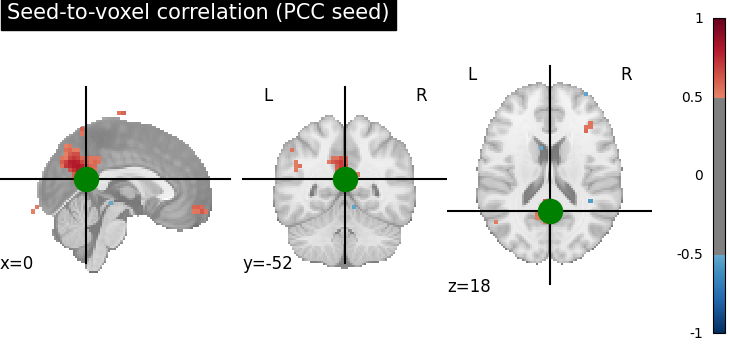# Producing single subject maps of seed-to-voxel correlation#

This example shows how to produce seed-to-voxel correlation maps for a single subject based on movie-watching fMRI scans. These maps depict the temporal correlation of a seed region with the rest of the brain.

This example is an advanced one that requires manipulating the data with numpy. Note the difference between images, that lie in brain space, and the numpy array, corresponding to the data inside the mask.

Author: Franz Liem

Note

If you are using Nilearn with a version older than `0.9.0`, then you should either upgrade your version or import maskers from the `input_data` module instead of the `maskers` module.

That is, you should manually replace in the following example all occurrences of:

```from nilearn.maskers import NiftiMasker
```

with:

```from nilearn.input_data import NiftiMasker
```

## Getting the data#

We will work with the first subject of the brain development fmri data set. dataset.func is a list of filenames. We select the 1st (0-based) subject by indexing with ).

```from nilearn import datasets

dataset = datasets.fetch_development_fmri(n_subjects=1)
func_filename = dataset.func
confound_filename = dataset.confounds
```

Note that func_filename and confound_filename are strings pointing to files on your hard drive.

```print(func_filename)
print(confound_filename)
```
```/home/yasmin/nilearn/nilearn_data/development_fmri/development_fmri/sub-pixar123_task-pixar_space-MNI152NLin2009cAsym_desc-preproc_bold.nii.gz
```

## Time series extraction#

We are going to extract signals from the functional time series in two steps. First we will extract the mean signal within the seed region of interest. Second, we will extract the brain-wide voxel-wise time series.

We will be working with one seed sphere in the Posterior Cingulate Cortex (PCC), considered part of the Default Mode Network.

```pcc_coords = [(0, -52, 18)]
```

We use `nilearn.maskers.NiftiSpheresMasker` to extract the time series from the functional imaging within the sphere. The sphere is centered at pcc_coords and will have the radius we pass the NiftiSpheresMasker function (here 8 mm).

The extraction will also detrend, standardize, and bandpass filter the data. This will create a NiftiSpheresMasker object.

```from nilearn.maskers import NiftiSpheresMasker

pcc_coords,
detrend=True,
standardize="zscore_sample",
low_pass=0.1,
high_pass=0.01,
t_r=2,
memory="nilearn_cache",
memory_level=1,
verbose=0,
)
```

Then we extract the mean time series within the seed region while regressing out the confounds that can be found in the dataset’s csv file

```seed_time_series = seed_masker.fit_transform(
func_filename, confounds=[confound_filename]
)
```
```/home/yasmin/miniconda3/envs/nilearn-dev/lib/python3.10/site-packages/joblib/memory.py:349: FutureWarning:

The default strategy for standardize is currently 'zscore' which incorrectly uses population std to calculate sample zscores. The new strategy 'zscore_sample' corrects this behavior by using the sample std. In release 0.13, the default strategy will be replaced by the new strategy and the 'zscore' option will be removed. Please use 'zscore_sample' instead.
```

Next, we can proceed similarly for the brain-wide voxel-wise time series, using `nilearn.maskers.NiftiMasker` with the same input arguments as in the seed_masker in addition to smoothing with a 6 mm kernel

```from nilearn.maskers import NiftiMasker

smoothing_fwhm=6,
detrend=True,
standardize="zscore_sample",
low_pass=0.1,
high_pass=0.01,
t_r=2,
memory="nilearn_cache",
memory_level=1,
verbose=0,
)
```

Then we extract the brain-wide voxel-wise time series while regressing out the confounds as before

```brain_time_series = brain_masker.fit_transform(
func_filename, confounds=[confound_filename]
)
```
```/home/yasmin/miniconda3/envs/nilearn-dev/lib/python3.10/site-packages/joblib/memory.py:349: FutureWarning:

The default strategy for standardize is currently 'zscore' which incorrectly uses population std to calculate sample zscores. The new strategy 'zscore_sample' corrects this behavior by using the sample std. In release 0.13, the default strategy will be replaced by the new strategy and the 'zscore' option will be removed. Please use 'zscore_sample' instead.
```

We can now inspect the extracted time series. Note that the seed time series is an array with shape n_volumes, 1), while the brain time series is an array with shape (n_volumes, n_voxels).

```print("Seed time series shape: (%s, %s)" % seed_time_series.shape)
print("Brain time series shape: (%s, %s)" % brain_time_series.shape)
```
```Seed time series shape: (168, 1)
Brain time series shape: (168, 32504)
```

We can plot the seed time series.

```import matplotlib.pyplot as plt

plt.plot(seed_time_series)
plt.title("Seed time series (Posterior cingulate cortex)")
plt.xlabel("Scan number")
plt.ylabel("Normalized signal")
plt.tight_layout()
```Exemplarily, we can also select 5 random voxels from the brain-wide data and plot the time series from.

```plt.plot(brain_time_series[:, [10, 45, 100, 5000, 10000]])
plt.title("Time series from 5 random voxels")
plt.xlabel("Scan number")
plt.ylabel("Normalized signal")
plt.tight_layout()
```## Performing the seed-to-voxel correlation analysis#

Now that we have two arrays (sphere signal: (n_volumes, 1), brain-wide voxel-wise signal (n_volumes, n_voxels)), we can correlate the seed signal with the signal of each voxel. The dot product of the two arrays will give us this correlation. Note that the signals have been variance-standardized during extraction. To have them standardized to norm unit, we further have to divide the result by the length of the time series.

```import numpy as np

seed_to_voxel_correlations = (
np.dot(brain_time_series.T, seed_time_series) / seed_time_series.shape
)
```

The resulting array will contain a value representing the correlation values between the signal in the seed region of interest and each voxel’s signal, and will be of shape (n_voxels, 1). The correlation values can potentially range between -1 and 1.

```print(
"Seed-to-voxel correlation shape: (%s, %s)"
% seed_to_voxel_correlations.shape
)
print(
"Seed-to-voxel correlation: min = %.3f; max = %.3f"
% (seed_to_voxel_correlations.min(), seed_to_voxel_correlations.max())
)
```
```Seed-to-voxel correlation shape: (32504, 1)
Seed-to-voxel correlation: min = -0.642; max = 0.954
```

## Plotting the seed-to-voxel correlation map#

We can now plot the seed-to-voxel correlation map and perform thresholding to only show values more extreme than +/- 0.5. Before displaying, we need to create an in memory Nifti image object. Furthermore, we can display the location of the seed with a sphere and set the cross to the center of the seed region of interest.

```from nilearn import plotting

seed_to_voxel_correlations.T
)
display = plotting.plot_stat_map(
seed_to_voxel_correlations_img,
threshold=0.5,
vmax=1,
cut_coords=pcc_coords,
title="Seed-to-voxel correlation (PCC seed)",
)
marker_coords=pcc_coords, marker_color="g", marker_size=300
)
# At last, we save the plot as pdf.
display.savefig("pcc_seed_correlation.pdf")
```## Fisher-z transformation and save nifti#

Finally, we can Fisher-z transform the data to achieve a normal distribution. The transformed array can now have values more extreme than +/- 1.

```seed_to_voxel_correlations_fisher_z = np.arctanh(seed_to_voxel_correlations)
print(
"Seed-to-voxel correlation Fisher-z transformed: "
f"min = {seed_to_voxel_correlations_fisher_z.min():.3f}; "
f"max = {seed_to_voxel_correlations_fisher_z.max():.3f}f"
)
```
```Seed-to-voxel correlation Fisher-z transformed: min = -0.762; max = 1.874f
```

Eventually, we can transform the correlation array back to a Nifti image object, that we can save.

```seed_to_voxel_correlations_fisher_z_img = brain_masker.inverse_transform(
seed_to_voxel_correlations_fisher_z.T
)
seed_to_voxel_correlations_fisher_z_img.to_filename(
"pcc_seed_correlation_z.nii.gz"
)
```

Total running time of the script: ( 0 minutes 17.731 seconds)

Estimated memory usage: 708 MB

Gallery generated by Sphinx-Gallery